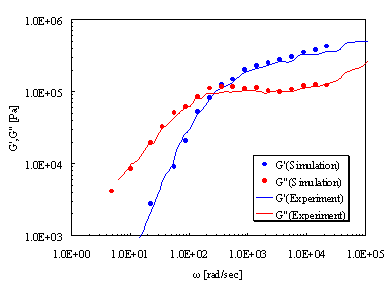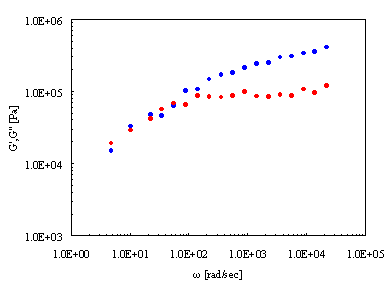Category
• Engine -
• NAPLES
• Materials -
• Plastics, Rubbers/Elastomers

# Rheological Characteristics

By performing coarse-graining through the level of the molar weight between polymer chain entanglement points, we are able to calculate the movement of polymer chain entanglement over long periods of time. This enables the evaluation of rheology properties. Here we use NAPLES to analyze dynamic viscoelasticity.

Following Reference , we evaluated G' (storage elastic modulus) of polyisoprene (molecular weight of ca. 48800) and G" (loss elastic modulus). The simulation results based on calculations involving pre-quantified molecular weights between entanglement points are compared to the experimental data results. As Figure 1 shows, these results match up very well with the experimental data.

Additionally, a cross-link structure can also be input using NAPLES. Figure 2 shows the calculation results when 10% of the entanglement points within the system are changed to cross-link points. The graph confirms these changes (for low frequency domains, the elastic behavior improves considerably).Figure 1. Dynamic ViscoelasticityFigure 2. Dynamic Viscoelasticity and Cross-Link Results

• Reference
•  Y. Masubuchi, G. Ianniruberto, F. Greco, G. Marrucci, J. Non-Newtonian Fluid Mech., 149, 87-92, (2008)